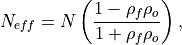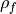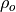# climpred.metrics._effective_sample_size#

climpred.metrics._effective_sample_size(forecast: xarray.Dataset, verif: xarray.Dataset, dim: Optional[Union[str, List[str]]] = None, **metric_kwargs: Any) [source]#

Effective sample size for temporally correlated data.

Note

Weights are not included here due to the dependence on temporal autocorrelation.

Note

This metric can only be used for hindcast-type simulations.

The effective sample size extracts the number of independent samples between two time series being correlated. This is derived by assessing the magnitude of the lag-1 autocorrelation coefficient in each of the time series being correlated. A higher autocorrelation induces a lower effective sample size which raises the correlation coefficient for a given p value.

The effective sample size is used in computing the effective p value. See _pearson_r_eff_p_value() and _spearman_r_eff_p_value().whereandare the lag-1 autocorrelation coefficients for the forecast and verification data.

Parameters

Notes

 minimum 0.0 maximum ∞ perfect N/A orientation positive

References

Bretherton et al. 

Example

>>> HindcastEnsemble.verify(
...     metric="effective_sample_size",
...     comparison="e2o",
...     alignment="same_verifs",
...     dim="init",
... )
<xarray.Dataset>
Coordinates:
* lead     (lead) int32 1 2 3 4 5 6 7 8 9 10
skill    <U11 'initialized'
Data variables:
SST      (lead) float64 5.0 4.0 3.0 3.0 3.0 3.0 3.0 3.0 3.0 3.0
Attributes: